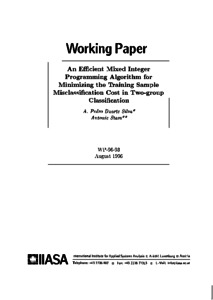# An Efficient Mixed Integer Programming Algorithm for Minimizing the Training Sample Misclassification Cost in Two-group Classification

Duarte Silva, A.P. & Stam, A. (1996). An Efficient Mixed Integer Programming Algorithm for Minimizing the Training Sample Misclassification Cost in Two-group Classification. IIASA Working Paper. IIASA, Laxenburg, Austria: WP-96-093Preview Text WP-96-093.pdf Download (1MB) | Preview

## Abstract

In this paper, we introduce the Divide and Conquer (D&C) algorithm, a computationally efficient algorithm for determining classification rules which minimize the training sample misclassification cost in two-group classification. This classification rule can be derived using mixed integer programming (MIP) techniques. However, it is well-documented that the complexity of MIP-based classification problems grows exponentially as a function of the size of the training sample and the number of attributes describing the observations, requiring special-purpose algorithms to solve even small size problems within a reasonable computational time. The D&C algorithm derives its name from the fact that it relies, a.o., on partitioning the problem in smaller, more easily handled subproblems, rendering it substantially faster than previously proposed algorithms.

The D&C algorithm solves the problem to the exact optimal solution (i.e., it is not a heuristic that approximates the solution), and allows for the analysis of much larger training samples than previous methods. For instance, our computational experiments indicate that, on average, the D&C algorithm solves problems with 2 attributes and 500 observations more than 3 times faster, and problems with 5 attributes and 100 observations over 50 times faster than Soltysik and Yarnold's software, which may be the fastest existing algorithm. We believe that the D&C algorithm contributes significantly to the field of classification analysis, because it substantially widens the array of data sets that can be analyzed meaningfully using methods which require MIP techniques, in particular methods which seek to minimize the misclassification cost in the training sample. The programs implementing the D&C algorithm are available from the authors upon request.

Item Type: Monograph (IIASA Working Paper) Institute Scholars (INS) IIASA Import 15 Jan 2016 02:07 27 Aug 2021 17:15 https://pure.iiasa.ac.at/4934View Item